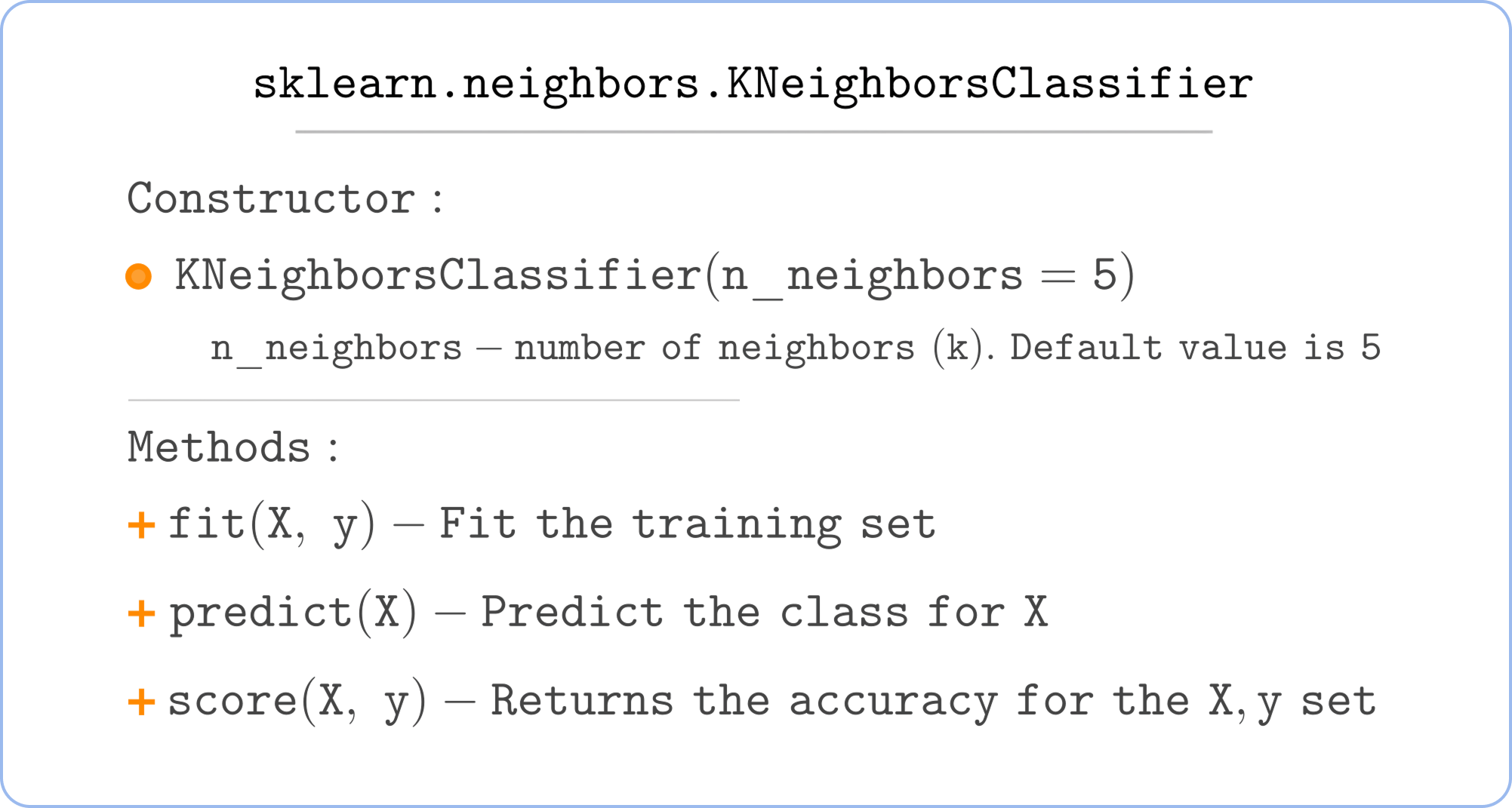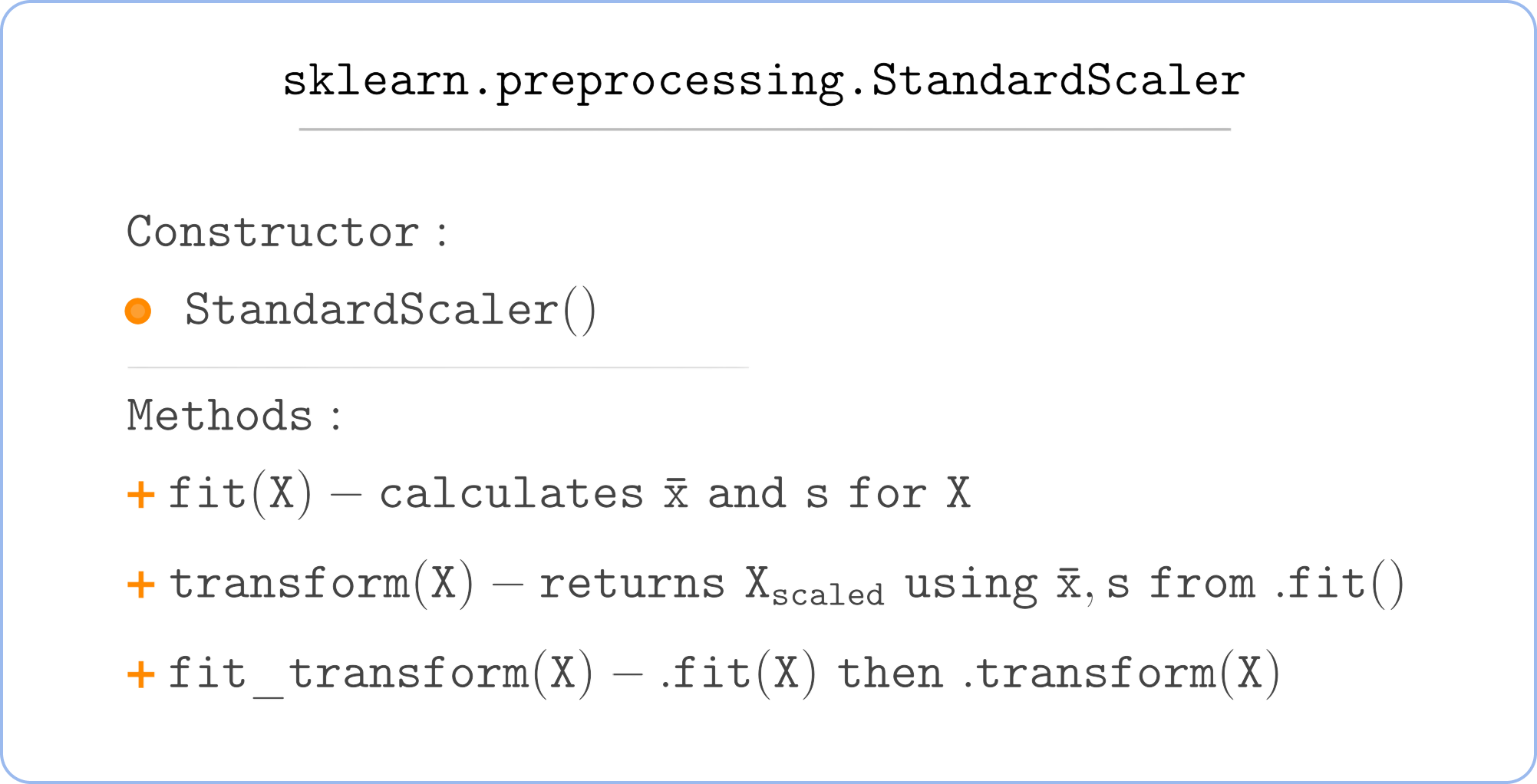Course Content

Classification with Python

##Implementing k-NN

### KNeighborsClassifier

Implementing k-Nearest Neighbors is pretty straightforward. We only need to import and use the `KNeighborsClassifier` class.Once you imported the class and created a class object like this:

You need to feed it the training data using the `.fit()` method:

And that's it! You can predict new values now.

### Scaling the data

However, remember that the data must be scaled. Let's take a closer look at the `StandardScaler` commonly used for scaling the data.
`StandardScaler` just subtracts the sample's mean and then divides the result by the sample's standard deviation.Note

If the terms sample mean and sample standard deviation sound unfamiliar, you can check out our Statistics course. But an understanding of what those numbers are for is not mandatory, so you can just ignore the meanings of and s and move on :)You should calculate and s on the training set(using either `.fit()` or `.fit_transform()`) and use the same and s to preprocess the data you are predicting(using `.transform()`). Here is an example:

If you use different x̄ and s for training set and new instances your predictions will likely be worse.

### Example

So to perform a k-NN classification in Python, you need to use `KNeighborsClassifier` and `StandardScaler`. Your code will look like this:

Here is a simple example where we try to predict whether the person will like Star Wars VI based on his ratings for Star Wars IV and V. We will read the data from the URL.The data is taken from The Movies Dataset with extra preprocessing. A person considered to like Star Wars VI if he/her rated it more than 4/5.

Everything was clear?

Section 1. Chapter 4